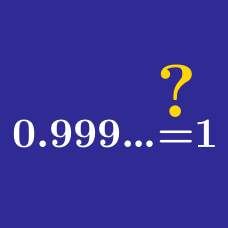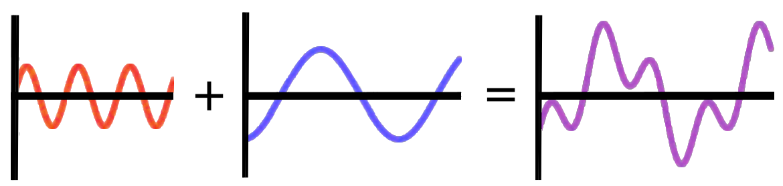Algebra

# Algebra Common Misconceptions: Level 2 Challenges

What is the value of

$\large \sqrt{ ( \color{#D61F06}-8\color{#333333}) ^ 2 } \hspace{.15cm} ?$

$\large {\color{#69047E}a}{\color{#D61F06}x} + {\color{#20A900}5}{\color{#69047E}b} = {\color{#D61F06}2}{\color{#69047E}a} + {\color{#69047E}b}{\color{#20A900}q} \\\\\\ \large \text{implies} \\\\\\ \large {\color{#D61F06}x} = {\color{#D61F06}2} \text{ and } {\color{#20A900}q}={\color{#20A900}5}.$

True or False?

For all real numbers ${\color{#69047E}a}, {\color{#69047E}b}, {\color{#D61F06}x}, \text{ and } {\color{#20A900}q}$, the statement above must be true.

\begin{aligned} \sqrt{-1}=&i \quad&\ldots(1) \\ \dfrac{1}{\sqrt{-1}}=&\dfrac{1}{i}\quad& \ldots(2) \\ \dfrac{\sqrt {1}}{\sqrt{-1}}=&\dfrac{1}{i} \quad &\ldots(3) \\ \sqrt{\dfrac{1}{-1}}=&\dfrac{1}{i} \quad &\ldots(4) \\ \sqrt{\dfrac{-1}{1}}=&\dfrac{1}{i}\quad &\ldots(5) \\ \dfrac{\sqrt{-1}}{1}=&\dfrac{1}{i} \quad &\ldots(6) \\ i=&\dfrac{1}{i} \quad& \ldots(7) \\ i^2=&1 \quad & \ldots (8) \\ -1=&1\quad & \ldots(9) \end{aligned}

Consider these steps above.

In which step is the (first) mistake committed?True or False:

Given any two periodic functions, $\color{#D61F06}{f(x)}$ and $\color{#3D99F6}{g(x)}$, the function $\color{#69047E}{h(x)} = \color{#D61F06}{f(x)} + \color{#3D99F6}{g(x)}$ will also be periodic with a period that is, at most, the product of the original two periods (the period of $f(x)$ times the period of $g(x)$).

What is the value of

$\large \sqrt{1\text{\%}}\, ?$

×